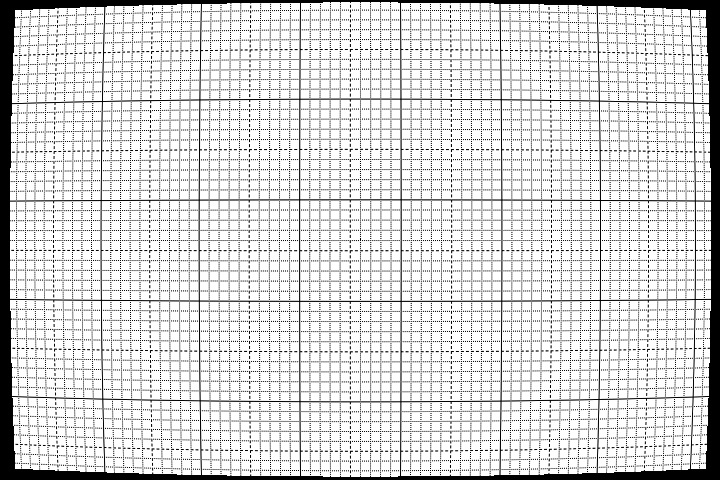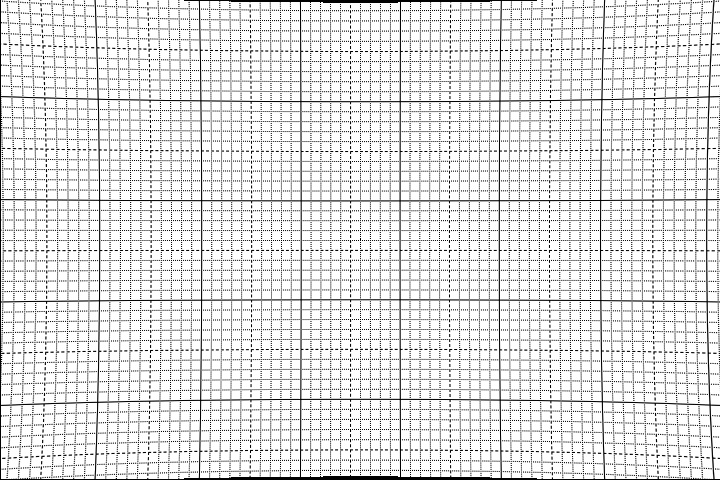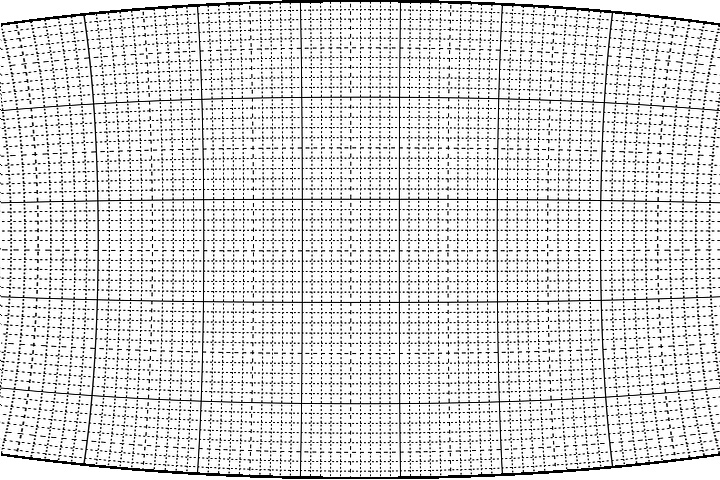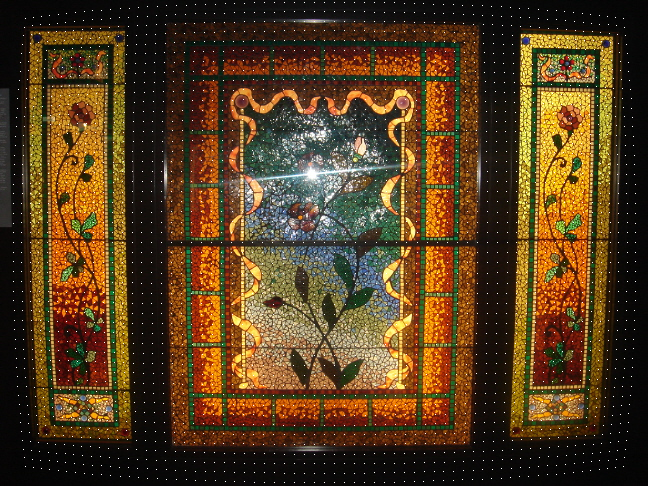DeBarrel

Author V. C. Mohan

This plugin corrects Barrel type or Pin Cushion type distortions in an image that result in due to camera lens projecting image on to a flat film or sensor array. ( The distortions due to perspective are not compensated by this plugin. For correcting perspective distortion one need to use Reform plugin.) This plugin can also correct for distortions if caused by factors independantly along x and y directions In the test mode a distorted matrix of dots is overlain on the specified frame

A polynomial with 4 coefficients are used to correct. a, b, and c are the coefficients to be specified and the fourth d is computed internally. These parameters mainly depend upon the lens of the camera, focal length of lens and setting of zoom.

For many high definition still photographic cameras databases are available on the web. But for video cams there is no such database. The possible reasons are 1. Video camera resolution is limited 2. The camera and or subjects move and therefore such distortions are not noticeable. However, due to advent of HD video cams and the consequent increased usage of tripod one may attempt to correct for such distortions.

By the use of test mode the values of coefficients can be arrived at. For a particular camera and setting of focus, these coefficients will remain same. In test mode the number of steps, the range of each of the coefficients etc. are specified. Then a clip with the selected frame of input clip as background is overlain with dots which lie along distorted lines. Each increment of output frame, these coefficients are altered by a step. This display may aid in selecting proper coefficients.

On some images unequal vertical and horizontal distortions are noticeable. For example if a frame based image is field separated, then the individual fields may exhibit such distortions.To be able to correct such distortion, a parameter vh can be used. This specifies the ratio of vertical to horizontal distortion.

Note a + b + c should not exceed 1.0 or less than - 1.0.
Input clip RGB and Y8 format only .
After correction one may need to crop image at the edges.
>Note. This Barrel or Pincushion distortion correction must be done on un cropped image or on symmetrically cropped image.
 Description Name Type Limits Default Input clip clip RGB or Y8 format only none coefficient a (xa in independant mode and start val in test mode) a float between - 0.5 and 0.5 0.005 coefficient b(xb in independant mode and start val in test mode) b float between - 0.5 and 0.5 0.005 coefficient c(xc in independant mode and start val in test mode) c float between - 0.5 and 0.5 0.005 whether to correct pin cushion or barrel type distortion pin Boolean true for pin, false for barrel false vertical to horizontal distortion ratio vh float between 0.1 and 10 1.0 whether in test mode test Boolean true for test, false for normal false end value of coefficient a in test mode(xea in independant mode) ea float between - 0.5 and 0.5 0.05 end value of coefficient b in test mode(xeb in independant mode) eb float between - 0.5 and 0.5 0.05 end value of coefficient c in test mode(xec in independant mode) ec float between - 0.5 and 0.5 0.05 number of steps for variation ( in test mode only) nsteps integer between 0 and 100 20 input frame number for over lay ( in test mode only) frame Int within input clip 0 color of dots in test mode and left out borders in normal color Int in RRGGBB format 0 whether x and y have independant coefficients ind Boolean true for independant, false for pin cushion or barrel false whether Y direction has pin cushion distortion? ypin Boolean true for pin cushion, false for barrel false value of coefficient ya ( start value in test mode ) ya float between - 0.5 and 0.5 0.05 value of coefficient yb (start value in test mode) yb float between - 0.5 and 0.5 0.05 value of coefficient yc (start value in test mode) yc float between - 0.5 and 0.5 0.05 end value of coefficient ya ( in test mode only) yea float between - 0.5 and 0.5 0.05 end value of coefficient yb ( in test mode only) yeb float between - 0.5 and 0.5 0.05 end value of coefficient yc ( in test mode only) yec float between - 0.5 and 0.5 0.05

#Usage examples:-
blankclip(width = 720, height = 480, pixel_type = "RGB32",color = \$ffffff,  length = 10)
grid()
pin = DeBarrel(a = 0.005, b = 0.005, c = 0.005,pin = false)
bar = DeBarrel(a = 0.005, b = 0.005, c = 0.005,pin = true)
test for image with independant distortions:-
DeBarrel(test=true,pin=false,  ind = true,a= 0.05,b= 0.011,c= 0.001, ea = .02, eb=0.012,ec= 0.001, ya= 0.1, yb= 0.011,yc= 0.001, yea= 0.01, yeb= 0.005, yec = 0.005 , ypin = true, color = \$ffffff)
Barrel Distortion Example:-Pin Cushion Distortion ExamplePin cushion and Barrel simultaneous. Use ind = true to correctwith following script dots displayed
DeBarrel(im,a = 0.005, b = 0.005, c = 0.005,pin = false, test = true, color = \$ffffff)>